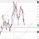5337 views
This creates a replica of relative distance from the moving averages, a good way to measure the strength, divergences etc.... short, mid and long term waves.

List of All my Indicators - https://www.tradingview.com/p/stocks/?so...
```study(title = "Momentum Histogram", shorttitle="Mom Hist")

source=close
EMA_1=input(13, title="EMA 1")
EMA_2=input(21, title="EMA 2")
EMA_3=input(34, title="EMA 3")

hist1 = (ema(close,EMA_1)*close)-(ema(close,EMA_2)*close)
hist2 = (ema(close,EMA_2)*close)-(ema(close,EMA_3)*close)

Wave1=(hist1)
Wave2=(hist2)

plot(Wave2, style=histogram, color=red, linewidth=4)
plot(Wave1, style=histogram, color=#0066cc, linewidth=4)```